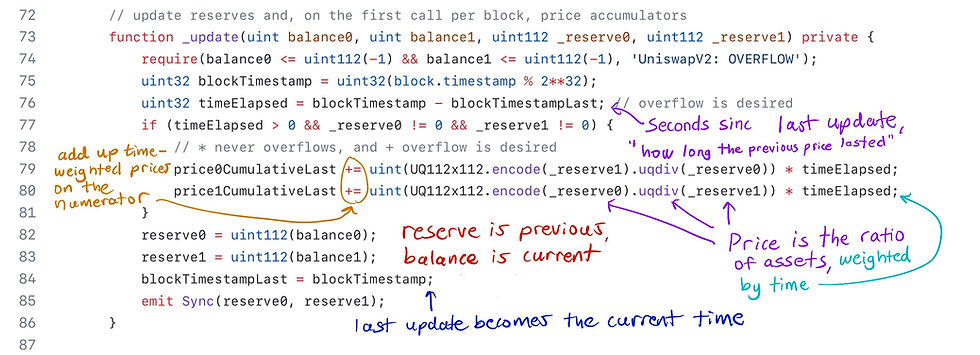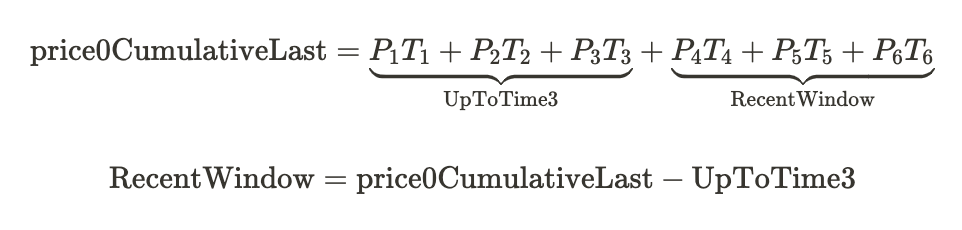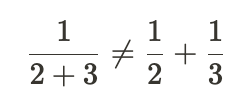top of page

# How the TWAP Oracle in Uniswap v2 Works

## What exactly is “price” in Uniswap?

Suppose we have 1 Ether and 2,000 USDC in a pool. This implies that the price of Ether is 2,000 USDC. Specifically, the price of Ether is 2,000 USDC / 1 Ether (ignoring decimals).

More generally, the price of an asset, in terms of the price of the other asset in the pair, is a ratio where the “asset you care about” is in the denominator.In the example above, it is saying “how may bars do you need to pay to get one foo” (ignoring fees).

## Price is a ratio

Because price is a ratio, they need to be stored with a data type which has decimal points (which Solidity types do not have by default).

That is, we say Ethereum is 2000 and USDC (in price of Ethereum) is 0.0005 (this is ignoring decimals of both assets).

Uniswap uses a fixed point number with 112 bits of precision on each side of the decimal, this takes up a total of 224 bits, and when packed with a 32 bit number, it uses up a single slot.

## Oracle definition

An oracle in computer science terms is a “source of truth.” A price oracle is a source of prices. Uniswap has an implied price when holding two assets, and other smart contracts can use this as a price oracle.

The intended users of the oracle are other smart contracts, since other smart contracts can easily communicate with Uniswap to determine the price, but getting price data from an off-chain exchange would be a lot harder.

However, just taking the ratio of the balances to get the current price isn’t safe.

## Motivation behind TWAP

Measuring an instantaneous snapshot of assets in the pool leaves an opportunity for flash loan attacks. That is, someone can make a huge trade using a flash loan to cause a temporary dramatic shift in the price, then take advantage of another smart contract that uses this price to make decisions.

The Uniswap V2 oracle defends against this in two ways:

1. It provides a mechanisms for consumers of the price (usually smart contracts) to take the average a previous time period (decided by the user). This means an attacker has to constantly manipulate the price for several blocks, which is a lot more costly than using a flash loan.

2. It doesn’t incorporate the current balance into the oracle calculation

This should not give the impression that oracles which use a moving average are immune to price manipulation attacks. If the asset does not have much liquidity, or the time window of taking the average is not sufficiently large, then a well-resourced attacker can still prop up the price (or suppress the price) long enough to manipulate the average price at the time of measurement.

## How TWAP works

A TWAP (Time Weighted Average Price) is like a simple moving average except that times where the price “stayed the same” longer get more weight — a TWAP weights price by how long the price stays at a certain level.

• Over the last day, the price of an asset was \$10 for the first 12 hours and \$11 for the second 12 hours. The average price is the same as the time weighted average price: \$10.5.

• Over the last day, the price of an asset was \$10 for the first 23 hours and \$11 for the most recent one. The expected average price should be closer to \$10 than \$11, but it will still be in between those values. Specifically, it will be (\$10 * 23 + \$11 * 1) / 24 = \$10.0417

• Over the last day, the price of an asset was \$10 for the first hour, and \$11 for the most recent 23 hours. We expect the TWAP to be closer to \$11 than 10. Specifically, it will be (\$10 * 1 + \$11 * 23) / 24 = \$10.9583

In general, the TWAP formula isHere T is a duration, not a timestamp. That is, how long the price stayed at that level.

## Uniswap V2 does not store lookback or the denominator

In our example above, we only looked at prices for the last 24 hours, but what if you care about prices for the last hour, week, or some other interval? Uniswap of course cannot store every look back that someone might be interested, and there also isn’t a good way to consistently snapshot the price as someone would have to pay for the gas.

The solution is that Uniswap only stores the numerator of values — every time a change is the liquidity ratio happens (mint, burn, swap, or sync are called), it records the new price and how long the previous price lasted.The variables price0Cumulativelast and price1CumulativeLast are public, so an interested party needs to snapshot them.

But this is an important point you should always remember price0CumulativeLast and price1CumulativeLast are only updated on lines 79 and 80 in the code above (orange circle), and they can only increase until they overflow. There is no mechanism make them “go down.” They always increase with every call to _update. This means they accumulate prices ever since the pool is launched, which could be a very long time.

## Limiting the lookback window

Clearly, we are generally not interested in the average price since the pool came into existence. We only want to look back a certain amount of time (1 hour, 1 day, etc).

Here is the TWAP formula gain.If we are only interested in prices since T4, then we want to be doing the followingHow do we accomplish this with code? Since the price0Cumulativelast keeps recordingWe need a way to isolate the parts we care about. Consider the followingIf we snapshot the price at the end of T3, we get the value UpToTime3. If we wait until T6 finishes, then we do price0Cumulativelast - UpToTime3then we will get the cumulative prices of only the recent window. If we divide that by the duration of the RecentWindow (T4 + T5 + T6), then we get the TWAP price of the recent window.

Graphically, this is what is what we are doing with the price accumulator.## Only calculating the last 1 hour TWAP in Solidity

If we want a 1 hour TWAP, we need to anticipate that we will need a snapshot of the accumulator one hour from now. So we need to access the public variable price0CumulativeLast and the public function getReserves() to get the last update time, and snapshot those values. (See the snapshot() function below).

After at least 1 hour has passed, we can call getOneHourPrice() and we will access the newest value of price0CumulativeLast from Uniswap V2.

Since the time we snapshotted the old price, Uniswap has been updating the accumulatorThe following code is made as simple as possible for illustration purposes, production use is not advised.### What if the last snapshot is over three hours ago?

Astute readers may note that the above contract will not be able to snapshot if the pair it is interacting with hasn’t had an interaction in the last three hours. The Uniswap V2 function _update is called during mint, burn, and swap, but none of those interactions happen, then lastSnapshotTime will record a time from a while ago. The solution is for the oracle to call the sync function at the time it does a snapshot, as that will internally call _update.

The sync function is screenshotted below.## Why TWAP must track two ratios

The price of A with respect to B is simply A/B and vice versa. For example, if we have 2000 USDC in the pool (ignoring decimals), and 1 Ether, then the price of 1 Ether is simply 2000 USDC / 1 ETH.

The price of USDC, denominated in ETH, is simply that number with the numerator and denominator flipped.

However, we cannot just “invert” one of the prices to get the other when we are accumulating pricing. Consider the following. If our price accumulator starts at 2 and adds 3, we cannot just do one over the accumulator:However, the prices are still “somewhat symmetric,” hence the choice of fixed point arithmetic representation must have the same capacity for the integers and for the decimals. If Eth is 1,000 times more “valuable” than a USDC, then USDC is 1,000 times “less valuable” than USDC. To store this accurately, the fixed point number should have the same size on both sides of the decimal, hence Uniswap’s choice of u112x112.

## PriceCumulativeLast always increases until it overflows, then keeps going

Uniswap V2 was built before Solidity 0.8.0, thus arithmetic overflowed and underflowed by default. Correct modern implementations of the price oracle need to use the unchecked block to ensure everything overflows as expected.

Eventually, the priceAccumulators and the block timestamp will overflow. In that case, the previous reserve will be higher than the new reserve. When the oracle computes the change in price, they will get a negative value. However, this won’t matter due to the rules of modular arithmetic.

To make things simple let’s use an imaginary unsigned integers that overflow at 100.

We snapshot the priceAccumulator at 80 and a few transactions/blocks later the priceAccumulator goes to 110, but it overflows to 10. We subtract 80 from 10, which gives -70. But the value is stored as an unsigned integer, so it gives -70 mod(100) which is 30. That’s the same result we would expect if it didn’t overflow (110-80=30).

This is true of all overflow boundaries, not just 100 in our example.

Overflowing the timestamp or priceAccumulator does not cause issues because of how modular arithmetic works.

### Overflowing the timestamp

The same thing happens when we overflow the timestamp. Because we are using a uint32 to represent it, there won’t be any negative numbers. Again, let’s assume we overflow at 100 for the sake of simplicity. If we snapshot at time 98 and consult the price oracle at time 4, then 6 seconds have passed. 4 - 98 % 100 = 6, as expected.